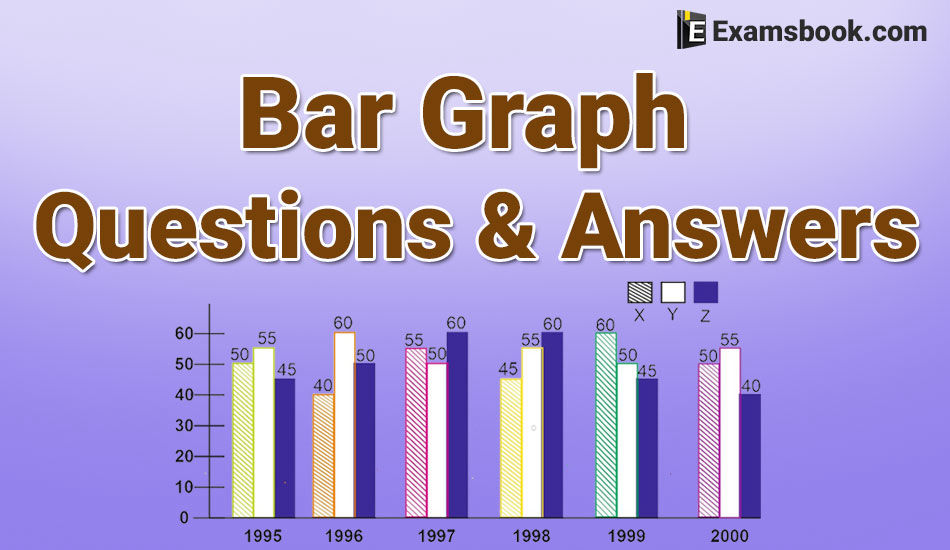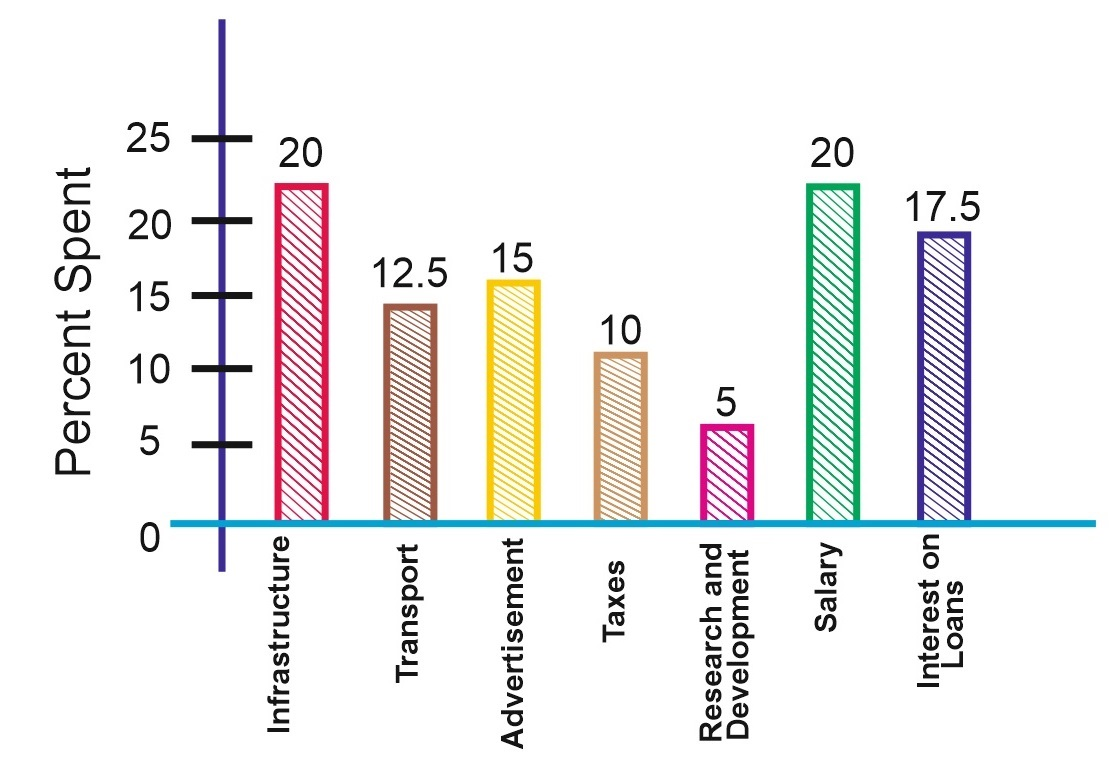• Save

Bar Graph Questions and Answers for Bank Exams

Last year 5.2K ViewsBar graph questions and answers are very important for the SSC and Bank exams. Generally, 2-3 bar graph questions asked in competitive exams but these 2-3 bar graph questions can improve your rank or score level in the examinations.

Here are the available important and selective bar graph questions and answers for all competitive exams. So, Students should try to solve these bar graph questions, for practice or improve their performance.

If you are preparing for any competitive exams like SSC, IBPS PO, Bank exam, etc. these Line graph questions with answersTabulation or table chart question-answers blogs will be very helpful for you.

You can read also: Pie chart questions and answers.

Bar Graph Questions and Answers for Competitive Exams

Direction (Question 1 to 5): The bar-graph given shows the percentage distribution of total expenditures of a company under various expense heads during 2003. Study the graph and answer the question that follows:

Percentage Distribution of Total Expenditure of a companyQ.1. The expenditures on the interest on loans are by what percent more than the expenditures on transport?

(A) 5%

(B) 10%

(C) 20%

(D) 30%

(E) 40%

Ans .  E

Q.2. What is the ratio of the total expenditure on infrastructure and transport to the total expenditure on taxes and interest on loans?

(A) 5: 4

(B)  8: 7

(C) 9: 7

(D) 13: 11

(E) Cannot be determined

Ans .  D

Q.3. If the expenditure on an advertisement is Rs. 2.10 crores then the difference between the expenditures on transport and taxes is:

(A) Rs. 1.25 crores

(B) Rs. 95 Lakhs

(C) Rs. 65 Lakhs

(D) Rs. 35 Lakhs

(E) Rs. 25 Lakhs

Ans .  D

Q.4.The total amount of expenditures of the company is how many times the expenditure on research and development?

(A) 27

(B) 20

(C) 18

(D) 8

(E) 5

Ans .  B

Q.5. If the interest on loans amounted to Rs. 2.45 cores then the amount of expenditure on advertisement, taxes and research and development is

(A) Rs. 7 crores

(B) Rs. 5.4 crores

(C) Rs. 4.2 crores

(D) Rs. 3 crores

(E) Rs. 2.4 crores

Ans .  C

Students can ask in comment section anything about bar graph questions and answers if they face any difficulty in bar graph questions and answers. Go to next page for more practice bar graph questions and answers for SSC and Bank Exams.